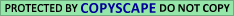## 3. Results and Conclusions

In Table 3, we list the rank order of each of the four Pi among the 1,000,000 corresponding Pip. Thus the entry 4 for P4means that for precisely 3 out of the 999,999 random permutations p, the statistic P4p was smaller than P4(none was equal). It follows that min ri = 0.000004 so r0 = 4 min ri = 0.000016. The same calculations, using the same 999,999 random permutations, were performed for control texts. Our first control text, R, was obtained by permuting the letters of G randomly (for details, see Section A.6 of the Appendix). After an earlier version of this paper was distributed, one of the readers, a prominent scientist, suggested to use as a control text Tolstoy's War and Peace. So we used text T consisting of the initial segment of the Hebrew translation of Tolstoy's War and Peace --of the same length of G. (TOLSTOY, L. N. War and Peace. Hebrew translation by L. Goldberg, Sifriat Poalim, Merhavia, 1953.)

Then we were asked by a referee to perform a control experiment on some early Hebrew text. He also suggested to use randomization on words in two forms: on the whole text and within each verse. In accordance, we checked texts I, U and W: text I is the Book of Isaiah; W was obtained by permuting the words of G randomly; U was obtained from G by permuting randomly words within each verse. In addition, we produced also text V by permuting the verses of G randomly. (For details, see Section A.6 of the Appendix.) Table 3 gives the results of these calculations, too. In the case of I, min ri is approximately 0.900; in the case of R it is 0.365; in the case of T it is 0.277; in the case of U it is 0.276; in the case of V it is 0.212; and in the case of W it is 0.516. So in five cases r0 = 4 min ri exceeds 1, and in the remaining case r0 = 0.847; that is, the result is totally nonsignificant, as one would expect for control texts. (FCAT (1986). The Book of Isaiah, file ISAIAH. MT. Facility for Computer Analysis of Texts (FCAT) and Tools for Septuagint Studies (CATSS), Univ. Pennsylvania, Philadelphia. (April 1986.) )

We conclude that the proximity of ELS's with related meanings in the Book of Genesis is not due to chance.

Table 3. Rank order of Pi among one million Piπ
P1 P2 p3 p4
G
453
5
570
4
R
619,140
681,451
364,859
573,861
T
748,143
363,481
580,307
277,103
I
899,830
932,868
929,840
946,261
W
883,770
516,098
900,642
630,269
U
321,071
275,741
488,949
491,116
V
211,777
519,115
410,746
591,503